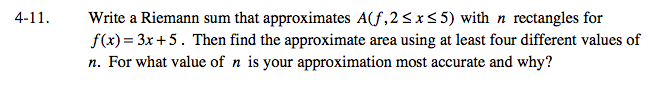### Home > CALC > Chapter 4 > Lesson 4.1.1 > Problem4-11

4-11.

Write a Riemann sum that approximates A(f, 2 ≤ x ≤ 5) with n rectangles for f(x) = 3x + 5. Then find the approximate area using at least four different values of n. For what value of n is your approximation most accurate and why? Homework Help ✎General form of left-endpoint Riemann sum: